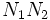# Normal join-closed group property

This article defines a group metaproperty: a property that can be evaluated to true/false for any group property
View a complete list of group metaproperties

## Definition

### Symbol-free definition

A group property$p$ is said to be normal join-closed or N-closed if it satisfies the following. Whenever there are two normal subgroups of a group, both having the property as abstract groups, then their join also has the property as an abstract group.

### Definition with symbols

A group property$p$ is said to be normal join-closed or N-closed if whenever$N_1, N_2 \triangleleft G$ such that$N_1$ and$N_2$ both satisfy$p$ as abstract groups, so does$N_1N_2$.